# 《趣学音视频》这段“朋友圈模版视频”的扛鼎之作是如何诞生的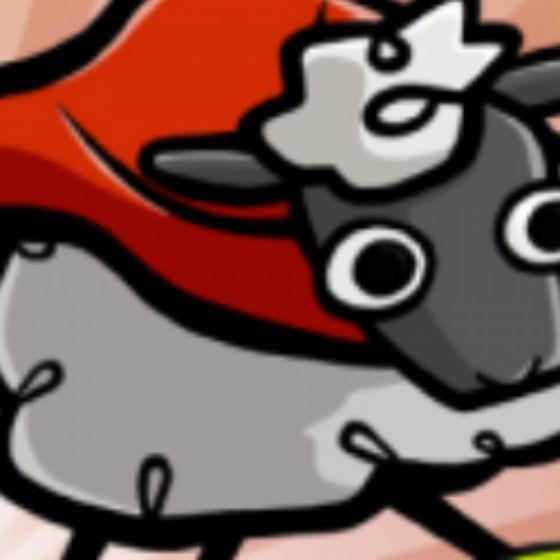ucsheep“长大”意味着什么？上面的视频给出了一个内涵且搞笑的答案。你看懂视频，摇摇头，会心一笑之时，是不是也有一丝伤怀——你已经和纯真、稚嫩彻底 Bye-Bye。既然已经看到这篇文章，不妨让我告诉你：一个长大的工程师，也是时候学点音视频知识了。

# 1.功能分析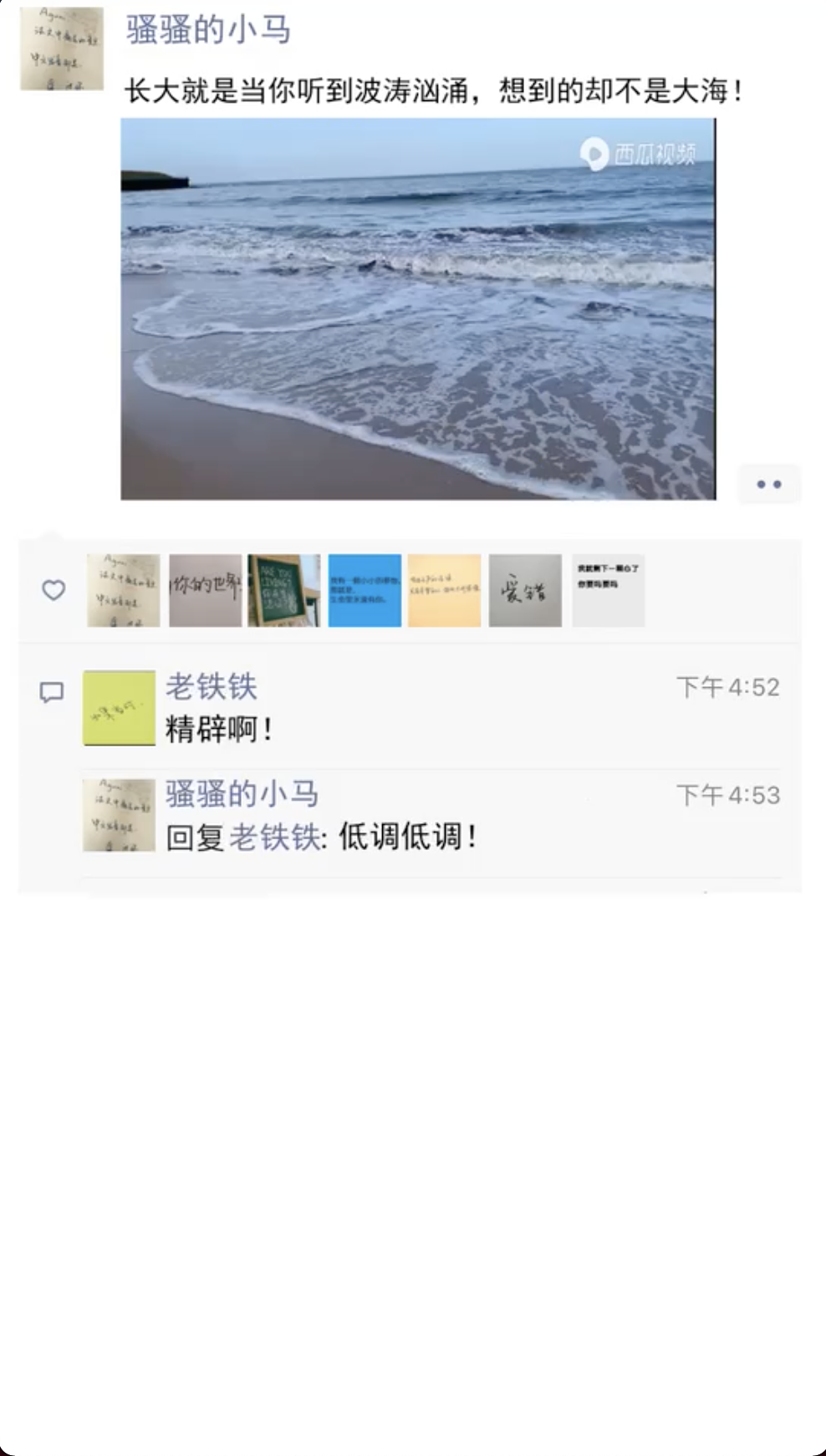1. 发布者昵称

2. 视频上方对应文案

3. 评论者昵称

4. 评论者对应文案

5. 发布者回复评论者的文案

6. 画面中心视频素材

## 1.2 程序流程及输出

1. 随机角色（1 个发布者+1 个评论者+7 个点赞者）分配头像图片

2. 渲染角色头像文案生成背景图片

3. 背景图片视频素材合成最终视频

# 3.实现步骤

## 3.1 基础底图制作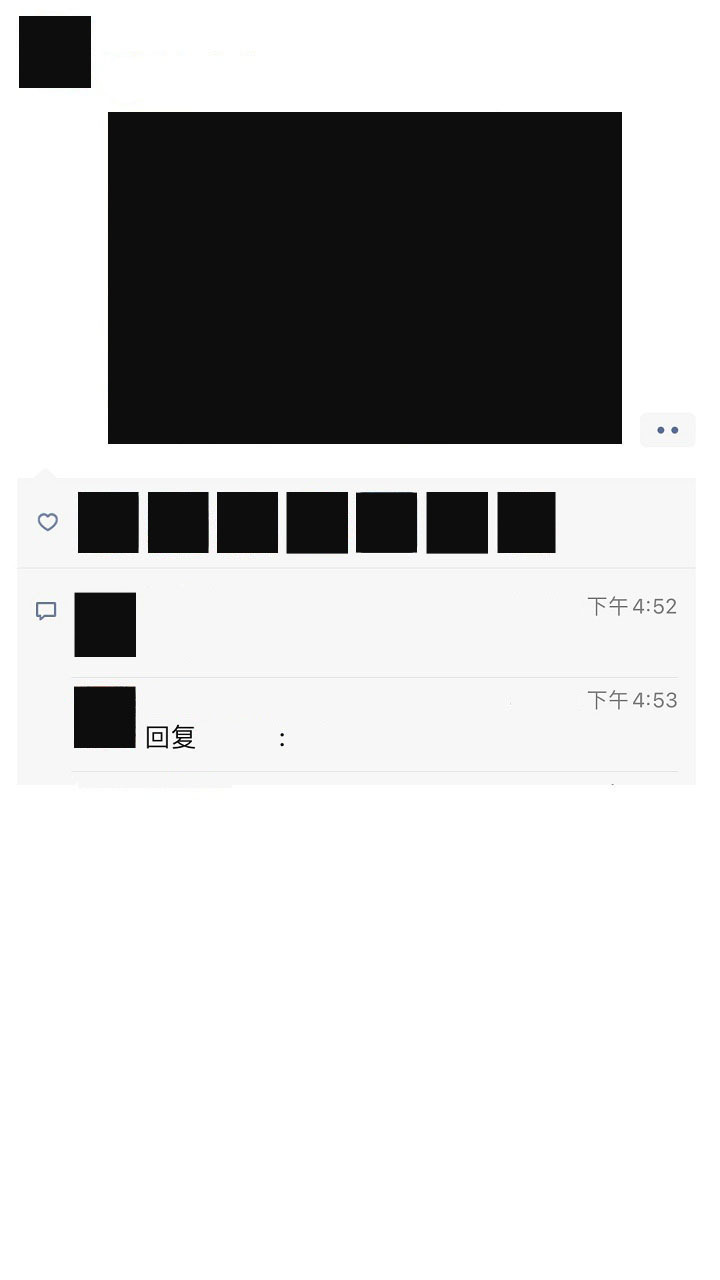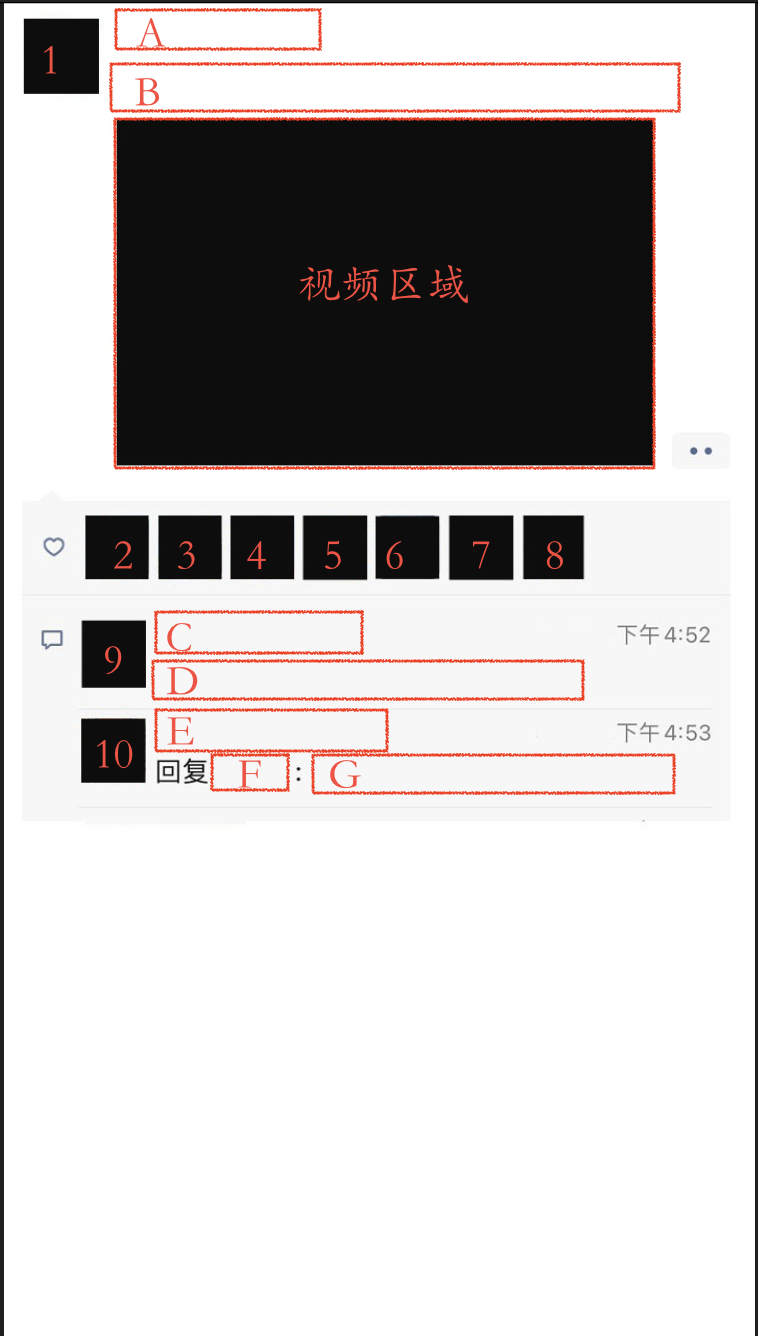• 图片类区域图片中用阿拉伯数字标注的 1-10 黑色区域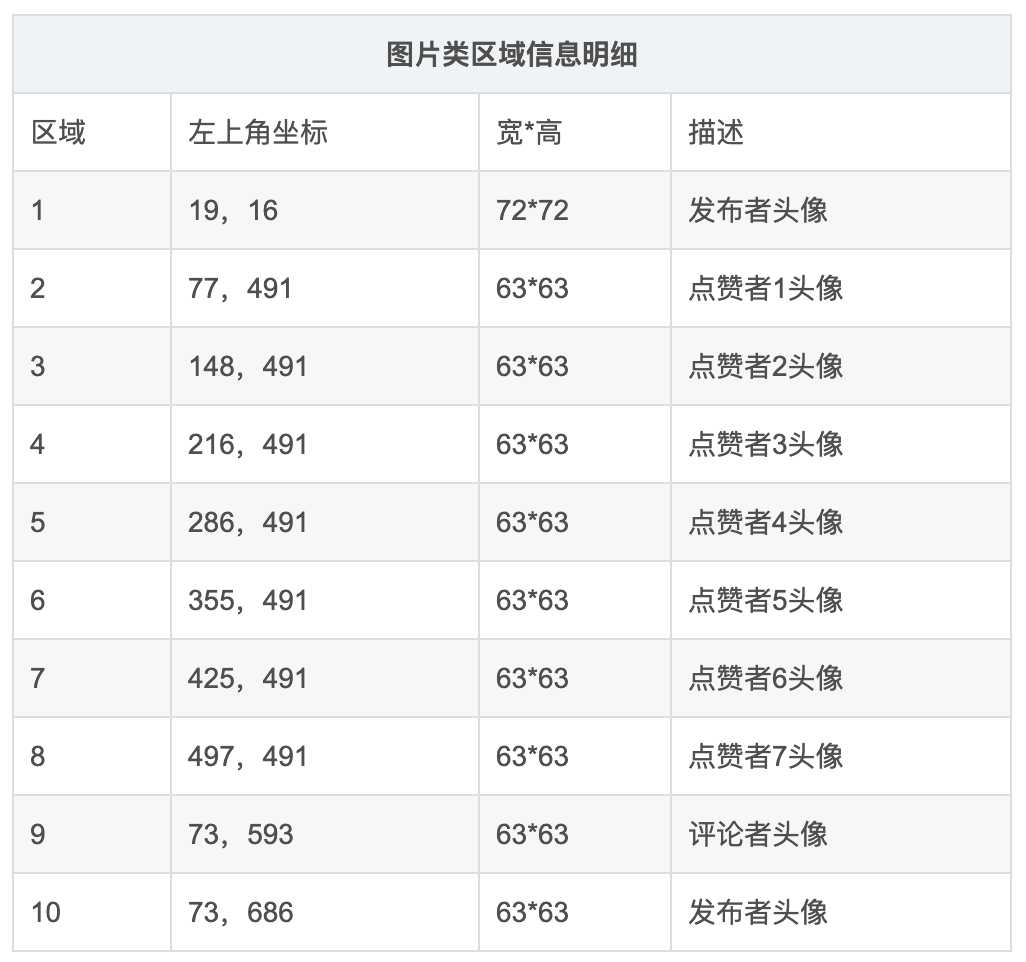• 文本类区域图片中使用红框框起并使字母 A-G 的区域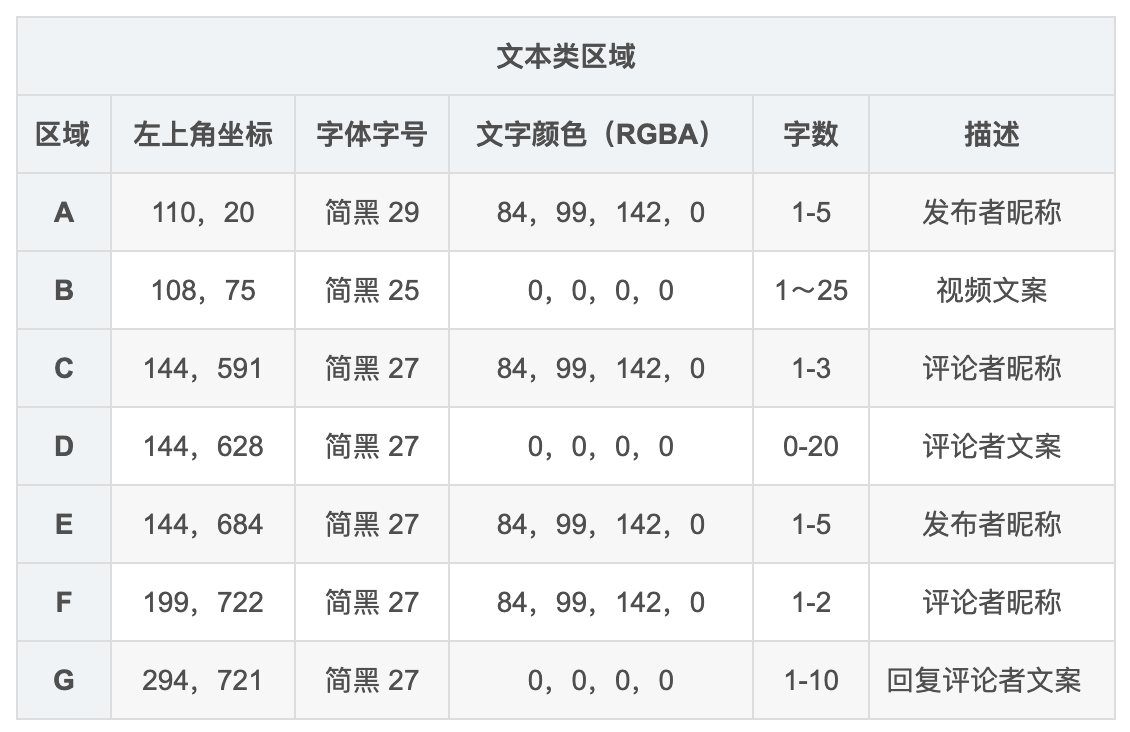• 视频区域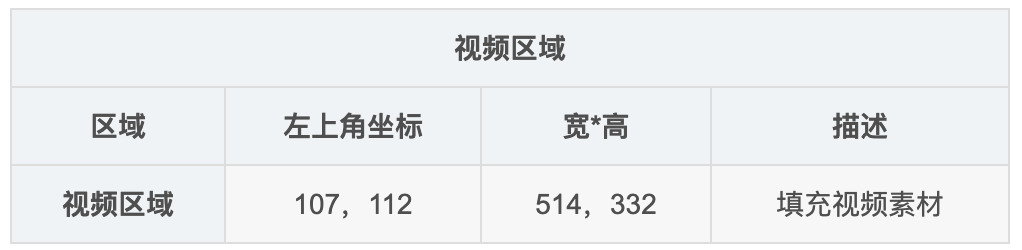## 3.2 背景图合成

### 3.2.1 头像渲染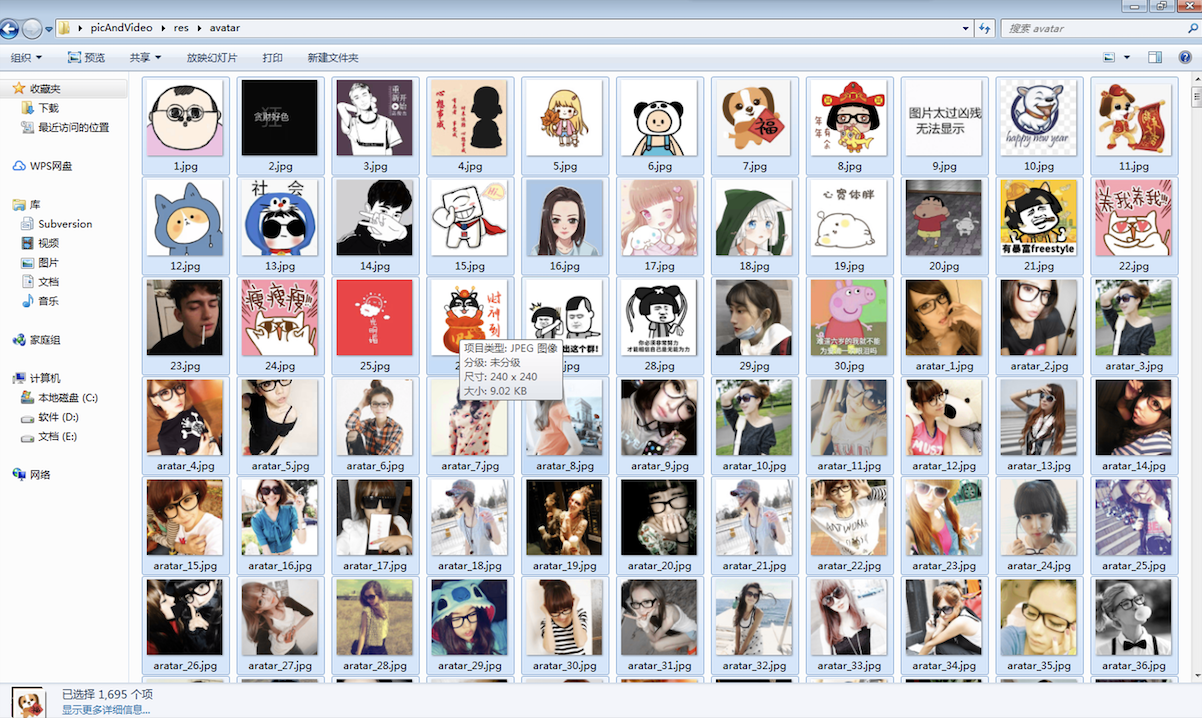def getAvatarRandom():    totalList = os.listdir("res/avatar")    if len(totalList) == 9:        return totalList    randIndex  = random.randint(0,len(totalList)-9)    return totalList[randIndex:randIndex+9]

# 载入基础底图bg_image = Image.open('res/bg.jpg')# 载入头像图片1avatar1 = Image.open('res/avatar/1.jpg')# 缩放头像图片至指定尺寸avatar1.thumbnail((72, 72))# 在基础底图上的指定区域渲染头像图片1bg_image.paste(avatar1, (19, 16))# 预览合成效果bg_image.show(bg_image)# 将合成效果保存为图片bg_image.save(fileName)

    # 载入底图    bg_image = Image.open('res/bg.jpg')  	# 随机获取9个头像并载入    aList = getAvatarRandom()    avatar1 = Image.open('res/avatar/' + aList)    avatar2 = Image.open('res/avatar/' + aList)    avatar3 = Image.open('res/avatar/' + aList)    avatar4 = Image.open('res/avatar/' + aList)    avatar5 = Image.open('res/avatar/' + aList)    avatar6 = Image.open('res/avatar/' + aList)    avatar7 = Image.open('res/avatar/' + aList)    avatar8 = Image.open('res/avatar/' + aList)    avatar9 = Image.open('res/avatar/' + aList)    # 渲染9个头像    avatar1.thumbnail((72, 72))    bg_image.paste(avatar1, (19, 16))    avatar1.thumbnail((63, 63))    bg_image.paste(avatar1, (73, 686))    avatar2.thumbnail((63, 63))    bg_image.paste(avatar2, (77, 491))    avatar3.thumbnail((63, 63))    bg_image.paste(avatar3, (148, 491))    avatar4.thumbnail((63, 63))    bg_image.paste(avatar4, (216, 491))    avatar5.thumbnail((63, 63))    bg_image.paste(avatar5, (286, 491))    avatar6.thumbnail((63, 63))    bg_image.paste(avatar6, (355, 491))    avatar7.thumbnail((63, 63))    bg_image.paste(avatar7, (425, 491))    avatar8.thumbnail((63, 63))    bg_image.paste(avatar8, (497, 491))    avatar9.thumbnail((63, 63))    bg_image.paste(avatar9, (73, 593))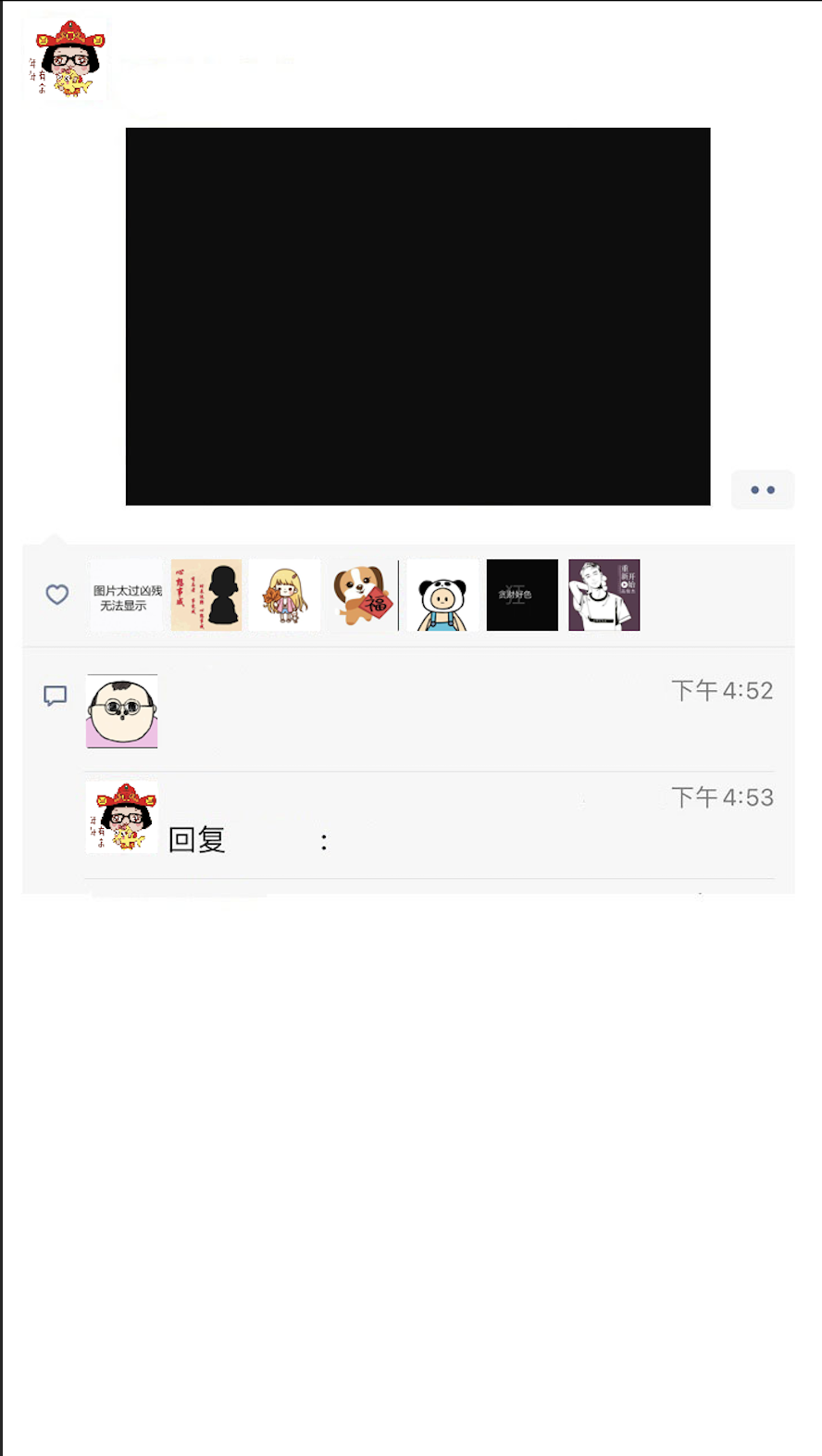### 3.2.2 文本渲染

# 生成一个画板draw = ImageDraw.Draw(bg_image)text1 = "长大就是当你听到波涛汹涌，想到的却不是大海！"# 载入样式font1 = ImageFont.truetype("res/simhei.ttf", size=25)# 使用draw在画板下面上指定区域，使用指定文字样式渲染文字draw.text(xy=(108, 75), text=text1, font=font1, fill=(0, 0, 0, 0))

    # 文本声明    text1 = "长大就是当你听到波涛汹涌，想到的却不是大海！"    text2 = "骚骚的小马"    text3 = "老铁铁"    text4 = "精辟啊！"    text5 = "低调低调！"    # 创建画板    draw = ImageDraw.Draw(bg_image)    # 渲染文本    font1 = ImageFont.truetype("res/simhei.ttf", size=25)    draw.text(xy=(108, 75), text=text1, font=font1, fill=(0, 0, 0, 0))    font2 = ImageFont.truetype("res/simhei.ttf", size=29)    draw.text(xy=(110, 20), text=text2, font=font2, fill=(84, 99, 142, 0))    font3 = ImageFont.truetype("res/simhei.ttf", size=27)    draw.text(xy=(144, 591), text=text3, font=font3, fill=(84, 99, 142, 0))    draw.text(xy=(199, 722), text=text3, font=font3, fill=(84, 99, 142, 0))    draw.text(xy=(144, 684), text=text2, font=font3, fill=(84, 99, 142, 0))    font4 = ImageFont.truetype("res/simhei.ttf", size=27)    draw.text(xy=(144, 628), text=text4, font=font4, fill=(0, 0, 0, 0))    font5 = ImageFont.truetype("res/simhei.ttf", size=27)    draw.text(xy=(294, 721), text=text5, font=font5, fill=(0, 0, 0, 0))    # 存储最终图片    bg_image.save("tmp/" + fileName)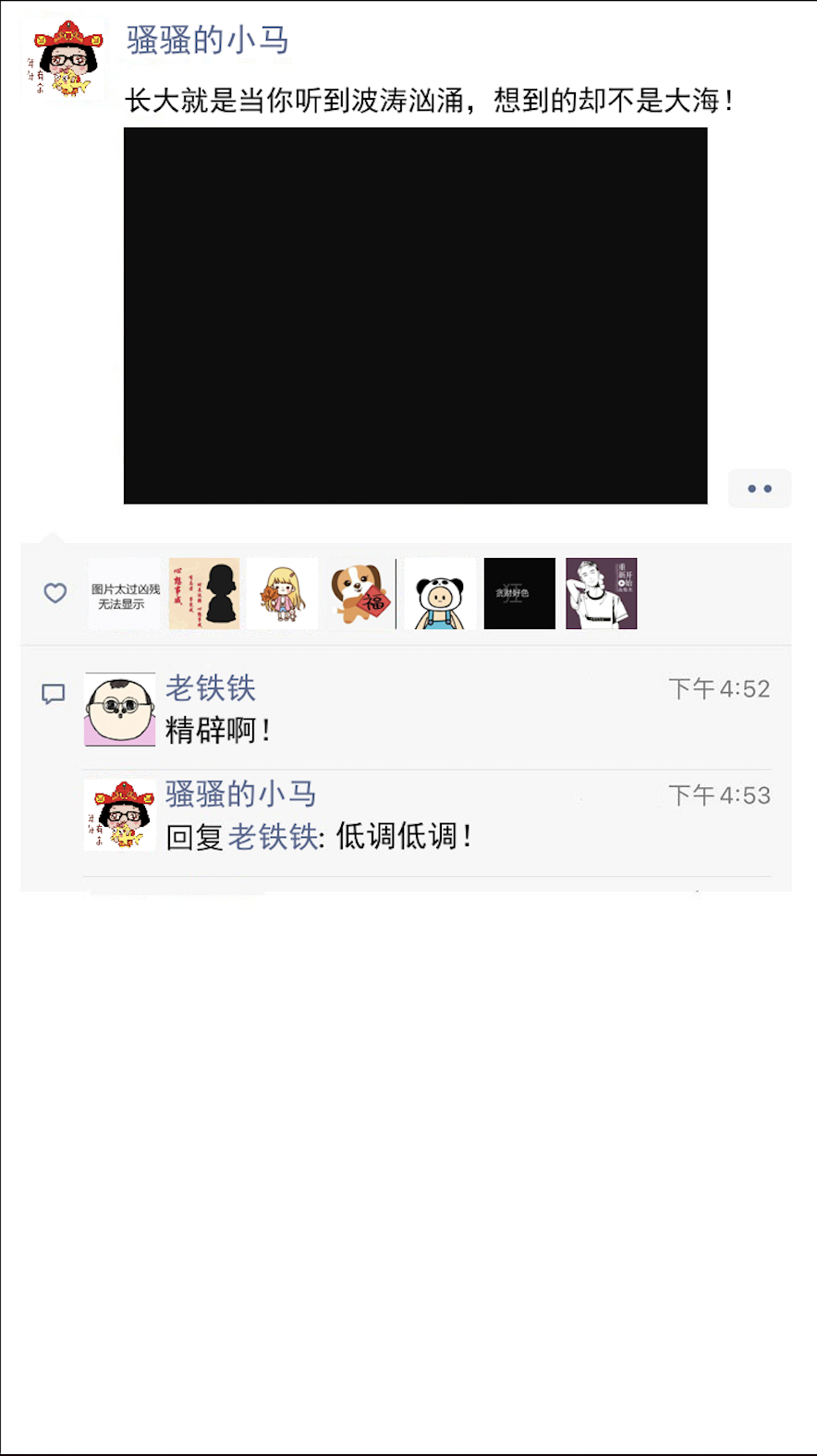nao~,目前就只剩下画面正中的视频区域了。

## 3.3 背景图与视频合成

ffmpeg.exe -i task_bg.png -vf "movie=demo.mp4,scale=514x332[test]; [in][test] overlay=x=107:y=112 [out]" output.mp4 

• demo.mp4 为准备的视频素材

• scale 对视频素材进行了缩放处理

• overlayx、y 指定了视频素材渲染的位置

cd 【视频素材路径】&&【ffmpeg所在目录】\ffmpeg.exe ……

# 4.结语

print("想要源码和程序嘛？关注后私信我，我会酌情给予（仅限学习目的）～")You see！Sheep！

## 评论